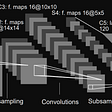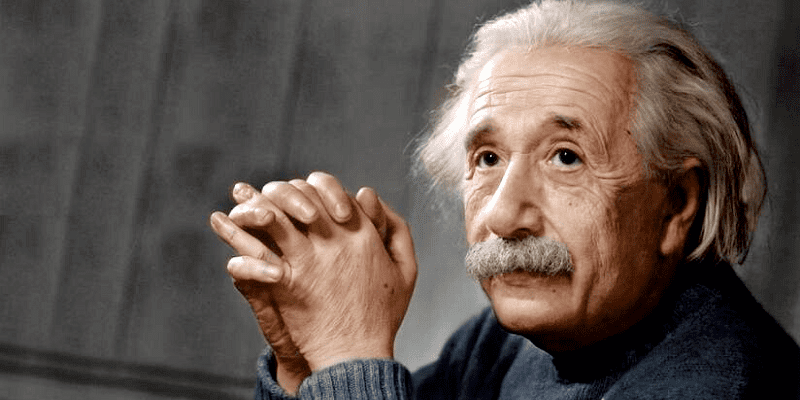# A Better Deep QA Tool

Retrieval of context based sole-ly on keywords is not doing it for me. I want something bigger, something better, something more intelligent. On my second out of 100 days of code, I created a contrastive Question-Knowledge encoding model, based on CLIP, using pytorch.

(big props to Moein’s article that I use a lot of code from here, about making a simplified version of CLIP: https://towardsdatascience.com/simple-implementation-of-openai-clip-model-a-tutorial-ace6ff01d9f2 , my code at the bottom of this article)

if you’re just here to use the tool, installation is as such.

`!pip install git+https://github.com/aicrumb/CQKPimport cqkpmodel = cqkp.load_model() # you have to sign into wandb first timearticles = [    "Birds can eat all nuts other than peanuts",    "Birds are a group of warm-blooded vertebrates",]model.best_answer("What is a bird?", articles)# expected output:# "Birds are a group of warm-blooded vertebrate`

# CLIP? Contrastive?

In Moein’s words:

In a nutshell, this model learns the relationship between a whole sentence and the image it describes

CLIP is a model that creates 512 dimensional vectors that are similar between text and image pairs. You put in a picture of a cute dog and it will give you 512 numbers that are very very close to the same 512 numbers you get if you put in just the words “A cute dog.”

Contrastive learning is all about making sure similar concepts are encoded similarly, and concepts that are far apart are encoded far apart.

# Lets do this!

Like I said before this pulls mainly from someone else’s code, but I do make changes here and there in the architecture, and rewrite the training loop from scratch for this circumstance.

First we have two text encoders, one for questions and one for articles. We use DistilBERT as the first half of the encoder, we put the last hidden state into a feed forward* model and that’s our encoding.

*We create two projection heads to resize the vector from DistilBERT from 768 dimensions to 1024 dimensions. What’s up with that? We aren’t going to finetune the DistilBERT model a lot at all (none) so we need some way to differentiate the encoders, this is what our differentiation looks like.

`class ProjectionHead(nn.Module):    def __init__(        self,        embedding_dim,        projection_dim=1024,        dropout=0.1    ):        super().__init__()        self.projection = nn.Linear(embedding_dim, projection_dim)        self.gelu = nn.GELU()        self.fc = nn.Linear(projection_dim, projection_dim)        self.dropout = nn.Dropout(dropout)        self.layer_norm = nn.LayerNorm(projection_dim)        def forward(self, x):        projected = self.projection(x)        x = self.gelu(projected)        x = self.fc(x)        x = self.dropout(x)        x = x + projected        x = self.layer_norm(x)        return x`

We have one feed forward layer, this is what does our translation of shapes.

Then a GELU layer, which is just an activation function. Activation functions are used to introduce non-linearity into a model, and I could go into why that’s important for deep learning, but there’s plenty other results on the internet explaining why.

We have another feed forward layer! This one is just for another transformation of the numbers, the more of these we have the further it diverges from the result from DistilBERT.

Next there’s a dropout layer, this is only active while we’re training, and will randomly drop out some of the inputs.

Then a layer normalization which is described here (https://arxiv.org/abs/1607.06450), I’m getting bored of explaining what everything does

In short, the projection head is used to transform the question and the article embeddings to the same embedding space with the same dimensionality.

(paraphrased from https://keras.io/examples/nlp/nl_image_search/)

# Training Time

We need a loss function.

`logits = (text_embeddings @ image_embeddings.T) / self.temperatureimages_similarity = image_embeddings @ image_embeddings.Ttexts_similarity = text_embeddings @ text_embeddings.Ttargets = F.softmax(    (images_similarity + texts_similarity) / 2 * self.temperature, dim=-1)texts_loss = cross_entropy(logits, targets, reduction='none')images_loss = cross_entropy(logits.T, targets.T, reduction='none')loss =  (images_loss + texts_loss) / 2.0return loss.mean()`

Wow that’s a lot of confusing things, but we can simplify what it does into one sentence.

This will maximize the similarity between training pairs of articles and questions, while minimizing the similarity between unpaired..pairs of articles and questions.

We need data, I chose the SQuAD dataset, it’s a huge dataset of questions and answers, along with helpful text. We’re just gonna take the questions and helpful text.

`import pandas as pd csv = pd.read_csv("SQuAD_csv.csv")contexts = csv['context']questions = csv['question']dataset = [(k,q) for k,q in zip(contexts,questions)]dataloader = DataLoader(dataset, batch_size=8, shuffle=True)`

This bit of code will put the questions and contexts into pairs for the model to take in.

We need an optimizer.

`import itertoolsparams = [    {"params": model.text_encoder.parameters(), "lr": 1e-3},    {"params": model.question_encoder.parameters(), "lr": 1e-3},    {"params": itertools.chain(        model.question_projection.parameters(), model.text_projection.parameters()    ), "lr": 1e-3, "weight_decay": 1e-3}]​optimizer = torch.optim.AdamW(params, weight_decay=0.)`

Here we specify which parameters to train, at what learning rate, and create the optimizer.

`for step, batch in enumerate(tqdm(dataloader)):    batch = model.tokenizer(        list(batch), padding=True, truncation=True, max_length=model.max_length    )    batch = model.tokenizer(        list(batch), padding=True, truncation=True, max_length=model.max_length    )    loss = model(torch.tensor(batch['input_ids'],device=device),                 torch.tensor(batch['input_ids'],device=device),                 torch.tensor(batch['attention_mask'],device=device),                 torch.tensor(batch['attention_mask'],device=device))    optimizer.zero_grad()    loss.backward()    optimizer.step()`

This is going to go through and compute tokens (the numerical form of text) for each article and question, and feed them as tensors into the model (which will return the loss, it’s in the model’s definition)

Then we just backward and make the optimizer take a step forward, we’re training!

# Use

Now we have the model, what can we do with it? Since it can encode both articles and questions, we can do anything that requires comparing the two numerically. One operation would be computing the right article to answer a particular question, which with the script I include, can be done with one line

`import cqkpmodel = cqkp.load_model(download=True) # you'll have to sign into wandb the first timearticles = [    "Birds can eat all nuts other than the usual peanuts",    "Birds are a group of warm-blooded vertebrates constituting the class Aves",]model.best_answer("what is a bird?", articles)`

How was that implemented? Lets take a look in the model definition

`def score(self,questions,answers):        text_features = self.text_encoder(torch.tensor(answers['input_ids'], device=device),torch.tensor(answers['attention_mask'],device=device))        question_features = self.question_encoder(torch.tensor(questions['input_ids'],device=device),torch.tensor(questions['attention_mask'],device=device))                question_embeddings = self.question_projection(question_features)        text_embeddings = self.text_projection(text_features)        return question_embeddings, text_embeddings        def best_answer(self, question, answers):        q_tok = self.tokenize([question])        a_tok = self.tokenize(answers)        scores = self.score(q_tok,a_tok)        scores = [torch.nn.functional.cosine_similarity(scores, scores[i]).item() for i in range(len(scores))]        ind = scores.index(max(scores))        return (answers[ind], ind, max(scores))`

Here we make a function that can compute the embeddings for question and answer pairs, then a function to compute those, then sort them by cosine similarity (which is just a distance function). We pick the one with the highest similarity (“`max(scores)`") and return it, along with the index of it in the questions provided, and the score too!

I think calling `model.best_answer("what is a bird?", articles)` is way simpler than rewriting all that every time, and way more user friendly, if this was a personal project just for me I definitely would've used the messier version (probably a bad idea but I'm not organized).

Here’s a link to the script, I’d love to see what people use it for!

https://github.com/aicrumb/CQKP

--

--

--

## More from crumb

silly little projects for silly little people. she/they/xe/it

Love podcasts or audiobooks? Learn on the go with our new app.

## Facial Recognition — the easy way## GSoC 2019 Phase 2: Hyphenation & Alignment## Region — Based Convolutional Neural Network (RCNN)## LeNet-5 CNN Architecture## Facial Expression Recognition Model## Brain Tumor Segmentation in MRI## Deploy a Machine Learning onnx Model on AWS using FastApi and Docker.## crumb

silly little projects for silly little people. she/they/xe/it

## Huggingface Transformers/Bert Pytorch: Play and Serve## ResNet with Data Augmentation## Easily Deploy Python Computer Vision Models To The Web Using Hugging Face Spaces## Deploying AI Models on Edge using OpenVino